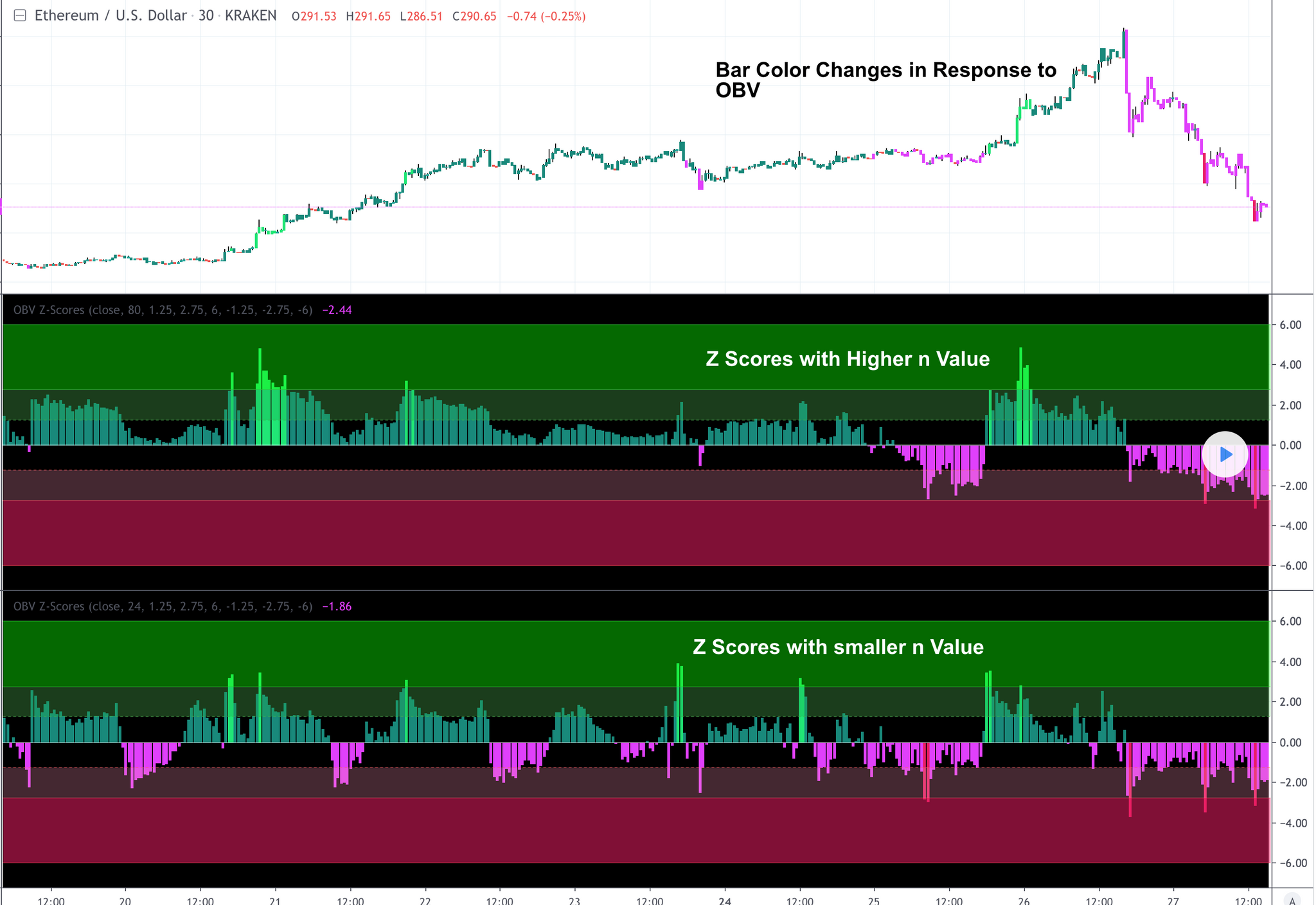Home / News / ETH/USD OBV Z-Scores New Script# ETH/USD OBV Z-Scores New Script

This study calculates the On-Balance Volume (OBV) and displays it in terms of its Z-Score.

OBV is a great momentum indicator . As the name suggests, OBV predicts changes in price based on the security's volume flow.

Formula:
if (Current Price > Previous Price)
then Current OBV = Previous OBV + Current Volume

if (Current Price < Previous Price)
then Current OBV = Previous OBV - Current Volume

if (Current Price == Previous Price)
then Current OBV = Previous OBV

As the formula shows, the OBV goes a step beyond just looking at the pure volume of a security. Instead, it factors in relative price action from period to period to reflect investor sentiment. As a result, we often look to the OBV to spot bullish or bearish trends while they are in the early stages of development or simply predict impending uptrends or downtrends.

To make the OBV easier to visualize, we converted the value to a Z-Score. The Z-Score is a simple statistical measurement and represents the current OBV value's distance from the mean OBV value in terms of # of standard deviations.

Users can adjust the values for the 2 upper bounds for Z Scores and 2 lower bounds. Additionally, the n value for z score calculation can be adjusted in the input menu. A higher n value means the z score will be based on a longer lookback period. A lower value will result in more sensitive readings.

Overall, I think this is an interesting way to represent OBV values and will be a valuable leading indicator.